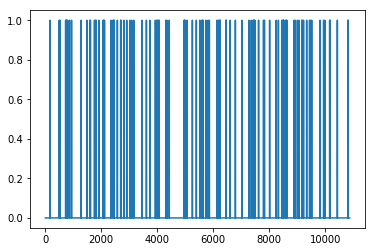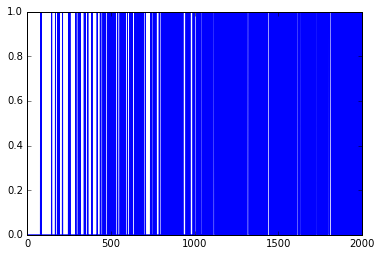2 views

Maybe my question will seem stupid.

I'm studying the Q-learning algorithm. To better understand it, I'm trying to remake the Tenzorflow code of this FrozenLake example into the Keras code.

My code:

import gym

import numpy as np

import random

from keras.layers import Dense

from keras.models import Sequential

from keras import backend as K

import matplotlib.pyplot as plt

%matplotlib inline

env = gym.make('FrozenLake-v0')

model = Sequential()

def custom_loss(yTrue, yPred):

return K.sum(K.square(yTrue - yPred))

model.compile(loss=custom_loss, optimizer='sgd')

# Set learning parameters

y = .99

e = 0.1

#create lists to contain total rewards and steps per episode

jList = []

rList = []

num_episodes = 2000

for i in range(num_episodes):

current_state = env.reset()

rAll = 0

d = False

j = 0

while j < 99:

j+=1

current_state_Q_values = model.predict(np.identity(16)[current_state:current_state+1], batch_size=1)

action = np.reshape(np.argmax(current_state_Q_values), (1,))

if np.random.rand(1) < e:

action = env.action_space.sample() #random action

new_state, reward, d, _ = env.step(action)

rAll += reward

jList.append(j)

rList.append(rAll)

new_Qs = model.predict(np.identity(16)[new_state:new_state+1], batch_size=1)

max_newQ = np.max(new_Qs)

targetQ = current_state_Q_values

targetQ[0,action] = reward + y*max_newQ

model.fit(np.identity(16)[current_state:current_state+1], targetQ, verbose=0, batch_size=1)

current_state = new_state

if d == True:

#Reduce chance of random action as we train the model.

e = 1./((i/50) + 10)

break

print("Percent of succesful episodes: " + str(sum(rList)/num_episodes) + "%")

When I run it, it doesn't work well: Percent of successful episodes: 0.052%

plt.plot(rList)The original Tensorflow code is much better: Percent of successful episodes: 0.352%

plt.plot(rList)What have I done wrong?

by (108k points)

You have to disable the bias like bias=False. Besides that, you can also try the trick in which you can start with the higher epsilon value. A trick might be to only decrease the epsilon value if you reach the goal. i.e. don't decrease epsilon at the end of every episode. That way your player can keep on exploring the map randomly, until it starts to converge on a good route, and then it'll be a good idea to reduce the epsilon parameter.